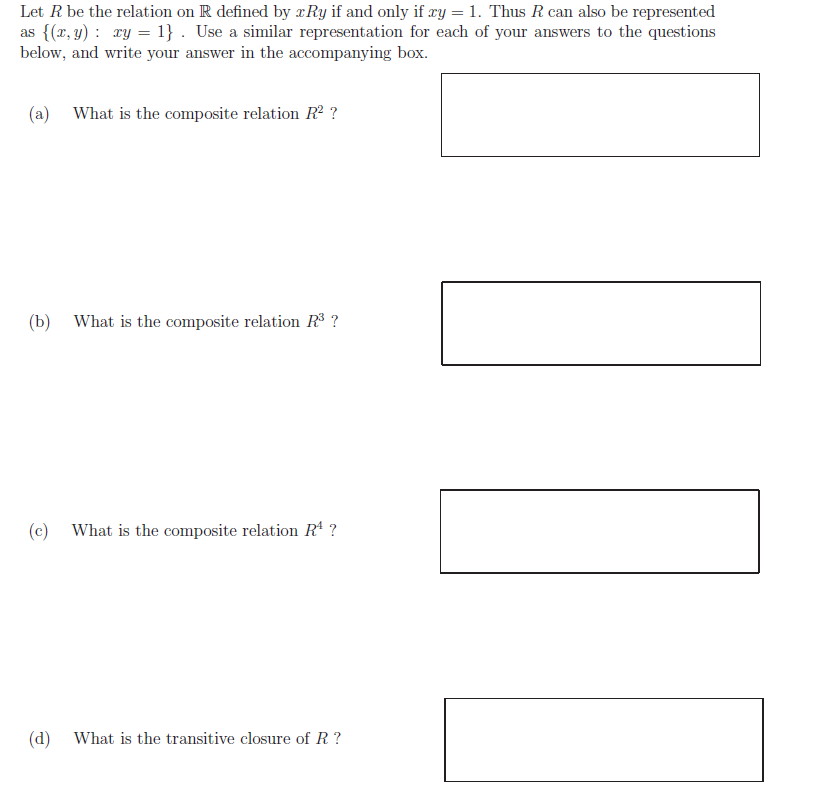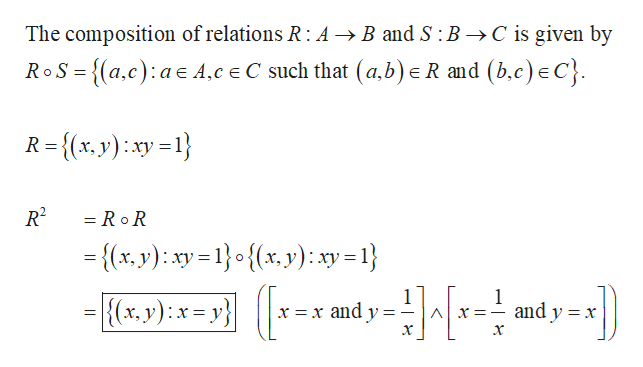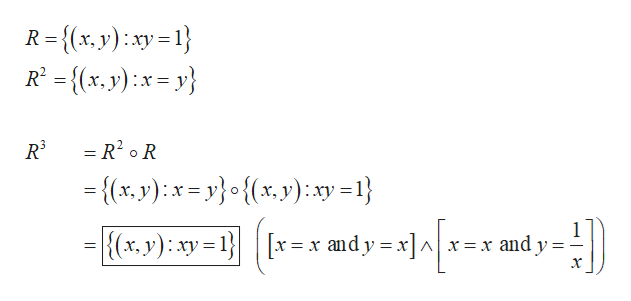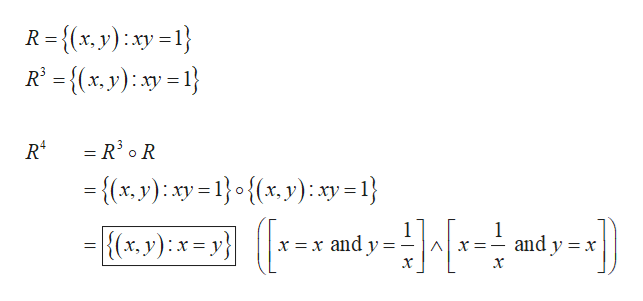# Let R be the relation on R defined by Ry if and only if ry 1. Thus R can also be representedas (r, y) ry = 1} . Use a similar representation for each of your answers to the questionsbelow, and write your answer in the accompanying boxWhat is the composite relation R2 ?(a)(b)What is the composite relation R3?What is the composite relation R4?(c)(d)What is the transitive closure of R?

Question
58 viewshelp_outlineImage TranscriptioncloseLet R be the relation on R defined by Ry if and only if ry 1. Thus R can also be represented as (r, y) ry = 1} . Use a similar representation for each of your answers to the questions below, and write your answer in the accompanying box What is the composite relation R2 ? (a) (b) What is the composite relation R3? What is the composite relation R4? (c) (d) What is the transitive closure of R? fullscreen
check_circle

star
star
star
star
star
1 Rating
Step 1

(a) Obtain the composite relation as follows.help_outlineImage TranscriptioncloseThe composition of relations R: A >B and S : B ->C is given by _ R and (b.c) eC} RoS-{a.c) a A.c e C such that (a.b) R={(x, y)xy R2 = RoR {(x, y): xy=1o{x, y): xy - 1} 1 x =x and y 1 and y x {(xr, y): x= y} X=_ =_ 11 х х fullscreen
Step 2

(b) Obtain the composite relation as follows.help_outlineImage TranscriptioncloseR= {(x, y):xy = 1} R² = {(x.y) :x = y} ху R = R? • R = {(x, y):x= y}•{(x.y):xy =1} х, |{(x.y): xy = 1}| [x = x and y = x]^ x =x and y fullscreen
Step 3

(c) Obtain the composite r...help_outlineImage TranscriptioncloseR ={(x,y) xyl} -{(x, y) sy = 1} = R3 o R -{x, y): ay-1o{(x, y) xy=1} { x. y):x= y} 1 and y x x =x and y X=_ fullscreen

### Want to see the full answer?

See Solution

#### Want to see this answer and more?

Solutions are written by subject experts who are available 24/7. Questions are typically answered within 1 hour.*

See Solution
*Response times may vary by subject and question.
Tagged in

### Math